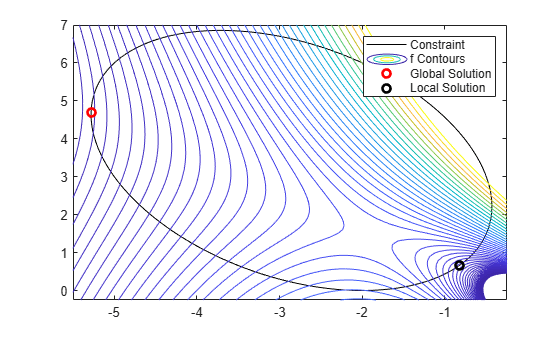# Solve Constrained Nonlinear Optimization, Problem-Based

This example shows how to find the minimum of a nonlinear objective function with a nonlinear constraint by using the problem-based approach. For a video showing the solution to a similar problem, see Problem-Based Nonlinear Programming.

To find the minimum value of a nonlinear objective function using the problem-based approach, first write the objective function as a file or anonymous function. The objective function for this example is

`$f\left(x,y\right)={e}^{x}\left(4{x}^{2}+2{y}^{2}+4xy+2y-1\right).$`

`type objfunx`
```function f = objfunx(x,y) f = exp(x).*(4*x.^2 + 2*y.^2 + 4*x.*y + 2*y - 1); end ```

Create the optimization problem variables `x` and `y`.

```x = optimvar('x'); y = optimvar('y');```

Create the objective function as an expression of the optimization variables.

`obj = objfunx(x,y);`

Create an optimization problem with `obj` as the objective function.

`prob = optimproblem('Objective',obj);`

Create a nonlinear constraint that the solution lies in a tilted ellipse, specified as

`$\frac{xy}{2}+\left(x+2{\right)}^{2}+\frac{\left(y-2{\right)}^{2}}{2}\le 2.$`

Create the constraint as an inequality expression of the optimization variables.

`TiltEllipse = x.*y/2 + (x+2).^2 + (y-2).^2/2 <= 2;`

Include the constraint in the problem.

`prob.Constraints.constr = TiltEllipse;`

Create a structure representing the initial point as `x = –3`, `y = 3`.

```x0.x = -3; x0.y = 3;```

Review the problem.

`show(prob)`
``` OptimizationProblem : Solve for: x, y minimize : (exp(x) .* (((((4 .* x.^2) + (2 .* y.^2)) + ((4 .* x) .* y)) + (2 .* y)) - 1)) subject to constr: ((((x .* y) ./ 2) + (x + 2).^2) + ((y - 2).^2 ./ 2)) <= 2 ```

Solve the problem.

`[sol,fval] = solve(prob,x0)`
```Solving problem using fmincon. Local minimum found that satisfies the constraints. Optimization completed because the objective function is non-decreasing in feasible directions, to within the value of the optimality tolerance, and constraints are satisfied to within the value of the constraint tolerance. ```
```sol = struct with fields: x: -5.2813 y: 4.6815 ```
```fval = 0.3299 ```

Try a different start point.

```x0.x = -1; x0.y = 1; [sol2,fval2] = solve(prob,x0)```
```Solving problem using fmincon. Feasible point with lower objective function value found. Local minimum found that satisfies the constraints. Optimization completed because the objective function is non-decreasing in feasible directions, to within the value of the optimality tolerance, and constraints are satisfied to within the value of the constraint tolerance. ```
```sol2 = struct with fields: x: -0.8210 y: 0.6696 ```
```fval2 = 0.7626 ```

Plot the ellipse, the objective function contours, and the two solutions.

```f = @objfunx; g = @(x,y) x.*y/2+(x+2).^2+(y-2).^2/2-2; rnge = [-5.5 -0.25 -0.25 7]; fimplicit(g,'k-') axis(rnge); hold on fcontour(f,rnge,'LevelList',logspace(-1,1)) plot(sol.x,sol.y,'ro','LineWidth',2) plot(sol2.x,sol2.y,'ko','LineWidth',2) legend('Constraint','f Contours','Global Solution','Local Solution','Location','northeast'); hold off```The solutions are on the nonlinear constraint boundary. The contour plot shows that these are the only local minima. The plot also shows that there is a stationary point near [–2,3/2], and local maxima near [–2,0] and [–1,4].

### Convert Objective Function Using `fcn2optimexpr`

For some objective functions or software versions, you must convert nonlinear functions to optimization expressions by using `fcn2optimexpr`. See Supported Operations for Optimization Variables and Expressions and Convert Nonlinear Function to Optimization Expression. Pass the `x` and `y` variables in the `fcn2optimexpr` call to indicate which optimization variable corresponds to each `objfunx` input.

`obj = fcn2optimexpr(@objfunx,x,y);`

Create an optimization problem with `obj` as the objective function just as before.

`prob = optimproblem('Objective',obj);`

The remainder of the solution process is identical.